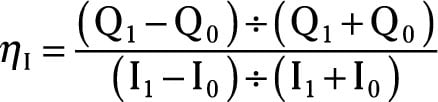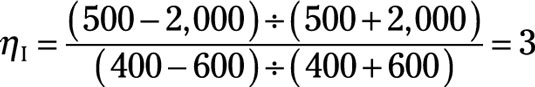##### Microeconomics For Dummies - UKCalculating the income elasticity of demand is essentially the same as calculating the price elasticity of demand, except you’re now determining how much the quantity purchase changes in response to a change in income.

The formula used to calculate the income elasticity of demand isThe symbol ηI represents the income elasticity of demand; η is the general symbol used for elasticity, and the subscript I represents income. In the formula, the symbol Q0 represents the initial demand or quantity purchased that exists when income equals I0. The symbol Q1 represents the new demand that exists when income changes to I1.

In this formula, the income elasticity of demand can be a positive or negative number, and it makes a real difference which it is. If the income elasticity of demand is negative, then the commodity is an inferior good. An inferior good is one whose demand decreases as incomes increase or demand increases as incomes decrease. (As an example, rice and potatoes are inferior goods.)

In other words, an inverse relationship exists between demand and income, and the income elasticity of demand is negative. This relationship is unusual.

The opposite situation is a normal good — normal because you get the expected or normal relationship. For a normal good, as income increases, the good’s demand increases. That’s what you expect, and most goods are normal. As your income increases, your demand for movie tickets, restaurant meals, cars, and maybe even asparagus increases. And the opposite will happen if your income decreases.

Therefore, normal goods have a direct relationship between income and demand, and the income elasticity of demand is positive.

Finally, the larger the number (either positive or negative) for the income elasticity of demand, the more responsive demand is to a change in income. A large number for the income elasticity of demand means a large change in demand occurs when income changes.

Say that you own a company that supplies vending machines. Currently, your vending machines sell soft drinks at \$1.50 per bottle, and at that price, customers purchase 2,000 bottles per week. For your community, the weekly income is \$600.

Then a major employer in the community closes, and a number of workers lose their job. The average weekly income in the community falls to \$400 and you note that your vending machine sales decrease to 500. You didn’t change the price of soft drinks, but your sales decreased dramatically due to the change in income.

The income elasticity of demand will tell you how responsive soft drink sales are to the change in income.

The method for calculating the income elasticity of demand is similar to the method used to calculate any elasticity. Here’s what you do:

1. Because \$600 and 2,000 are the initial income and quantity, put \$600 into I0 and 2,000 into Q0.

2. Because \$400 and 500 are the new income and quantity, put \$400 into I1 and 500 into Q1.

3. Start by dividing the expression on top of the equation.

(Q1 – Q0) equals –1,500, and (Q1 + Q0) equals 2,500. Dividing –1,500 by 2,500 equals –3/5.

4. Divide the expression in the bottom of the equation.

(I1 – I0) equals –\$200, and (I1 + I0) equals \$1,000. Dividing –\$200 by \$1,000 equals –1/5.

5. Divide the top result, –3/5, by the bottom result, –1/5.

You get the income elasticity of demand 3.

So the income elasticity of demand for soft drinks equalsThe income elasticity value tells you a 1 percent decrease in income causes a 3 percent decrease in demand. Thus, because you sell fewer soft drinks when incomes decrease, soft drinks are a normal good.